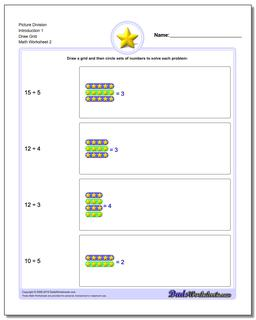# Math Worksheets: Picture Math Division: Picture Math Division: Picture Division Introduction 1 Draw Grid (Second Worksheet)## Picture Division Introduction 1 Draw Grid (Second Worksheet)

PropertyValue
DescriptionPicture Division Introduction 1 Draw Grid: Picture math division worksheets where the student must draw the dividend in a visual grid and then group it into corresponding sets in order to solve the problem. (Second Worksheet)
Resource TypeWorksheet